# Difference between revisions of "Artin-Schreier code"

Given an algebraic curve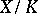, whereis a field of characteristic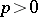, a covering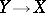is called an Artin–Schreier curve overif the corresponding extension of function fields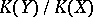is generated by some function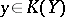such that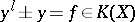(whereis a power of, cf. also Extension of a field). If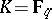is a finite field, it turns out that Artin–Schreier curves often have many rational points.

To be precise, let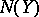(respectively,) denote the number of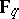-rational points (respectively, the genus) of a curve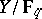. The Hasse–Weil theorem states thatIf the genus is large with respect to, this bound can be improved as follows. Let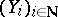be a sequence of curves oversuch that. ThenCurves overcan be used to construct error-correcting linear codes, so-called geometric Goppa codes or algebraic-geometric codes (cf. Error-correcting code; Goppa code; Algebraic-geometric code; [a4], [a5]). If the curves have sufficiently may rational points, these codes have very good error-correcting properties. Hence, one is interested in explicit constructions of curves with many rational points.

## Examples of Artin–Schreier curves.

The Hermitian curve over, for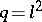, is given by the equation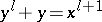. It has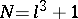rational points and its genus is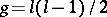. Hence, for it the Hasse–Weil boundis attained, see [a4].

Again, letbe a square. Define a tower of function fieldsover(cf. Tower of fields) by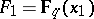,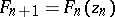, where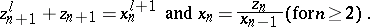For the corresponding algebraic curves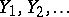, the coverings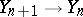are Artin–Schreier curves. This sequenceattains the Drinfel'd–Vladut bound, i.e.,(see [a1]).

The geometric Goppa codes constructed using these curves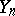beat the Gilbert–Varshamov bound (cf. also Error-correcting code; [a3]) for all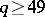. This construction is simpler and more explicit than the construction based on modular curves (the Tsfasman–Vladut–Zink theorem, [a5]).